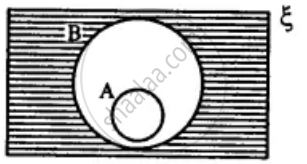# Draw a Venn-diagram to Show the Relationship Between Two Sets a and B; Such that a ⊆ B, Now Shade the Region Representing : (A ∪ B)' - Mathematics

Diagram

Draw a Venn-diagram to show the relationship between two sets A and B; such that A ⊆ B, Now shade the region representing :
(A ∪ B)'

#### Solution

(A ∪ B)' =Concept: Venn Diagram
Is there an error in this question or solution?

#### APPEARS IN

Selina Concise Mathematics Class 8 ICSE
Chapter 6 Sets
Exercise 6 (E) | Q 6.4 | Page 76